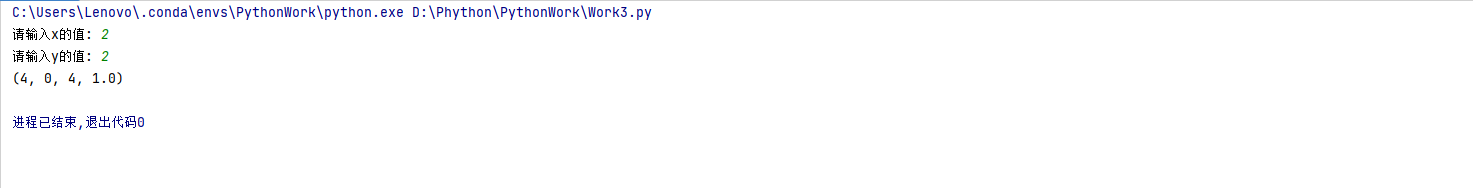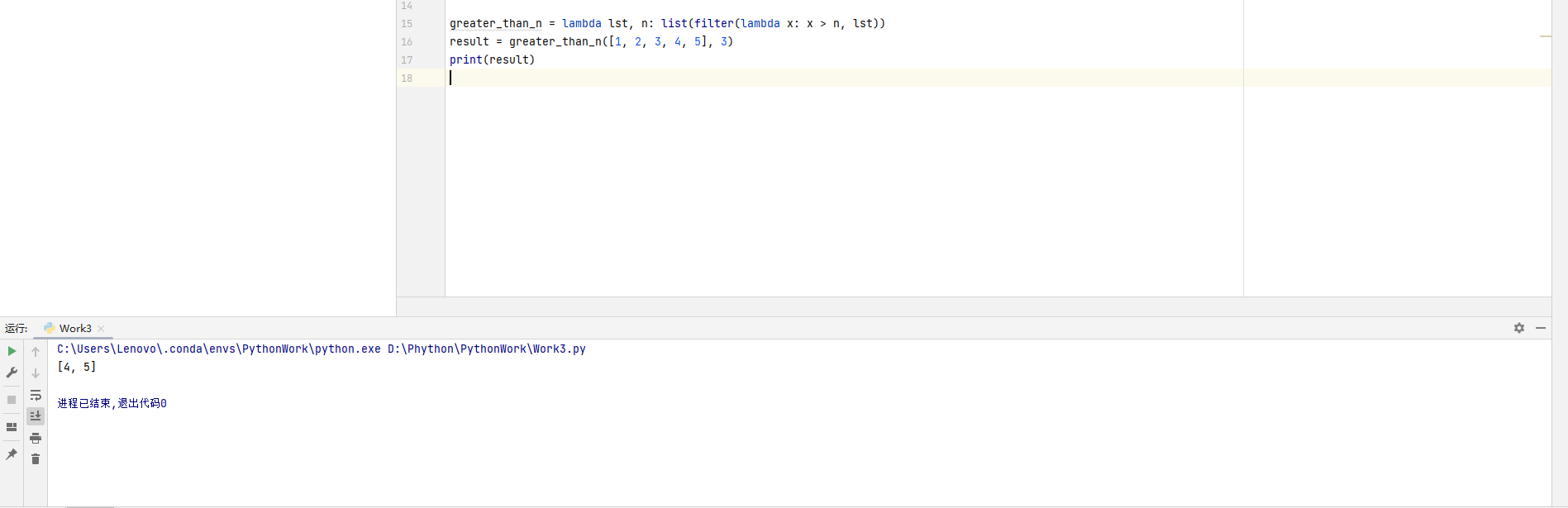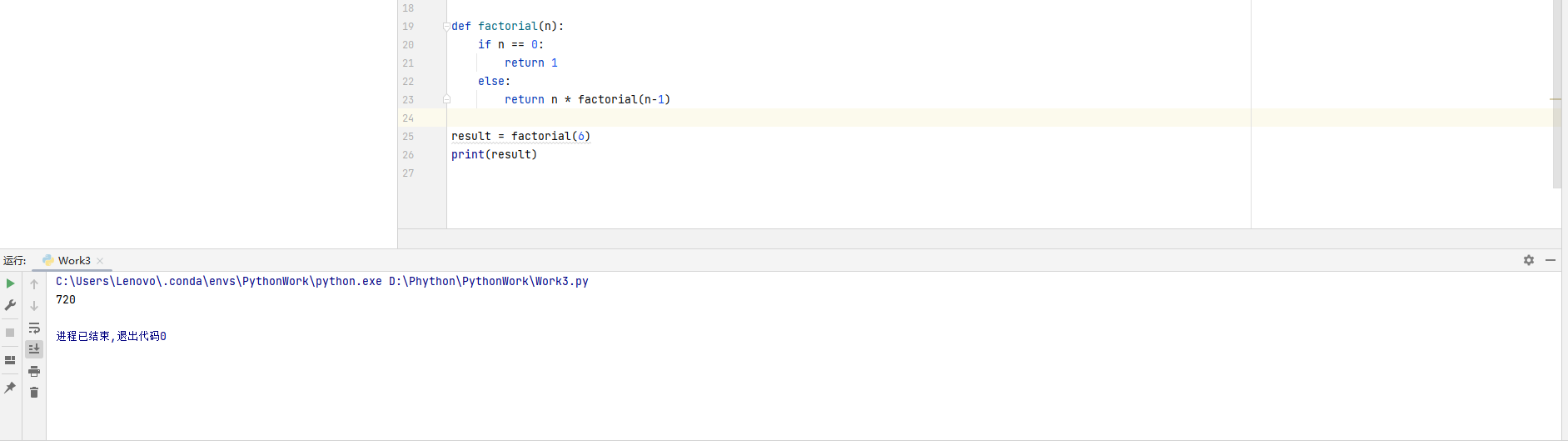# 2023（春）Python程序设计作业3： 函数及应用

Python编程的综合练习，涵盖了Python函数定义及使用、Lambda函数、递归函数、作用域等知识点：题目如下：

## 一 、函数定义及使用

### 输出

``````def calculate(x, y):
if y == 0:
return None
else:
sum = x + y
difference = x - y
product = x * y
quotient = round(x / y, 2)
return sum, difference, product, quotient
x = int(input('请输入x的值: '))
y = int(input('请输入y的值: '))
result = calculate(x, y)
print(result)``````## 二、Lambda函数

### 输出

``````greater_than_n = lambda lst, n: list(filter(lambda x: x > n, lst))
result = greater_than_n([1, 2, 3, 4, 5], 3)
print(result)``````## 三、递归函数

### 输出

``````def factorial(n):
if n == 0:
return 1
else:
return n * factorial(n-1)

result = factorial(6)
print(result)``````...全文
6 回复 打赏 收藏 转发到动态 举报Python可以这样学（第一季：Python内功修炼）

159发帖与我相关我的任务
python 高校 江苏省·南通市• 近7日
• 近30日
• 至今